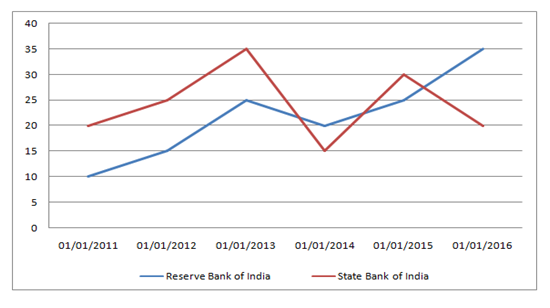# IBPS RRB PO Prelims Quantitative Aptitude Questions 2019 (Day-07)

Dear Aspirants, Our IBPS Guide team is providing new series of Quantitative Aptitude Questions for IBPS RRB PO Prelims 2019 so the aspirants can practice it on a daily basis. These questions are framed by our skilled experts after understanding your needs thoroughly. Aspirants can practice these new series questions daily to familiarize with the exact exam pattern and make your preparation effective.

Check here for IBPS RRB Prelims Mock Test 2019

[WpProQuiz 6635]

Directions (1 – 5): Study the following information carefully and answer the given questions.

Number of staffs (in thousands) in two different banks in six different years1) The number of staffs in Reserve Bank of India in the year 2015 is what Percentage increased as compared to the previous year?

a) 55

b) 25

c) 15

d) 45

e) 35

2) If 25% of the staffs in State Bank of India in the year 2014 were females, what was the number of male staffs in the State Bank of India in the same year?

a) 11250

b) 10750

c) 12650

d) 13250

e) 14950

3) In which year, the difference between the number of staffs in Reserve Bank of India and the number of staffs in State Bank of India is highest?

a) 2013

b) 2014

c) 2016

d) 2015

e) 2012

4) What was the difference between the average number of staffs in Reserve Bank of India and State Bank of India in all the years together?

a) 3000

b) 3500

c) 1500

d) 2500

e) 2000

5) What is the sum of the number of staffs in Reserve Bank of India in the year 2011, 2013 and 2015 together and the number of staffs in State Bank of India in the year 2012, 2014 and 2016 together?

a) 130000

b) 90000

c) 110000

d) 150000

e) 120000

Directions (6 – 10): What Approximate value should come in place of question mark (?) in the following questions?

6) 820.01 ÷ 21 × 2.99 + ? = 240

a) 105

b) 173

c) 123

d) 234

e) 143

7) 299 ÷ 12 × 13.95 + ? = (24.02)2

a) 285

b) 225

c) 325

d) 150

e) 185

8) 92 × 576 ÷ √1296 = (?)3 + √49

a) 3

b) 14

c) 11

d) 27

e) None of these

9) (21.5% of 999)(1/3) + (43% of 601)1/2 = ?

a) 18

b) 22

c) 26

d) 30

e) None of these

10)  40.005% of 439.998 +? % of 655.011 = 228.5

a) 8

b) 17

c) 12

d) 20

e) 5

Direction (1-5) :

Number of staffs in Reserve Bank of India in 2015 = 25000

Number of staffs in Reserve Bank of India in 2014 = 20000

Increase = 25000 – 20000 = 5000

Increase % = 5000/20000 × 100 = 25%

Total number of staffs in State Bank of India in 2014 = 15000

Number of females staffs = 25% of 15000

= 25/100 × 15000 = 3750

Number of male staffs = 15000 – 3750

= 11250

Difference between the numbers of staffs in Reserve Bank of India & Number of staffs in State Bank of India for the year

2011 – (20000 – 10000) = 10000

2012 – (25000 – 15000) = 10000

2013 – (35000 – 25000) = 10000

2014 – (20000 – 15000) = 5000

2015 – (30000 – 25000) = 5000

2016 – (35000 – 20000) = 15000

Difference was highest in year 2016

Average number of staffs in Reserve Bank of India = (10 + 15 + 25 + 20 + 25 + 35)/6

= 130/6

Average number of staffs in State Bank of India = (20 + 25 + 35 + 15 + 30 + 20)/6

= 145/6

Difference = (145/6) – (130/6)

= 15/6= 2.5*1000

= 2500

Number of staffs in Reserve Bank of India in 2011, 2013 and 2015

= 10000 + 25000 + 25000

= 60000

Number of staffs in State Bank of India in 2012, 2014 and 2016

= 25000 + 15000 + 20000

= 60000

Total staffs = 60000 + 60000

= 120000

Direction (6-10) :

820 ÷ 21 × 3 +? ≈ 240

Or, 39 × 3 +? ≈ 240

Or,? ≈ 240 – 117 = 123

? ≈ (24.02)2 – 299 ÷ 12 × 13.95

≈ 576 – 25 × 14 = 576 – 350 = 226 ≈ 225

(?)3 + √49 = 92 × 876 ÷ √1296

(?)3 + 7 = 92 × 576 ÷ 36

(?)3 + 7 = 92 × 576 ÷36

(?)3 = 1465

?= 11.3

? = (21.5 * 10)1/3 + (43 * 6)1/2

? = (215)(1/3) + (258)(1/2)

? = (63)(1/3) + (162)(1/2)

? = 6 + 16 = 22

40 * (440/100) + ?/100 * 655 = 228

4*44 + ? * 6.55 = 229

? = (229 – 176) / 7

? = 53/7 = 7.5 = 8